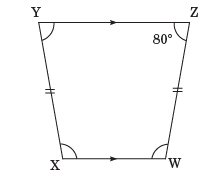# Understanding Quadrilaterals Class 8 Worksheets

## Understanding Quadrilaterals Class 8 Worksheets

Understanding Quadrilaterals Class 8 Worksheets play a vital role in strengthening the maths concepts. After studying the concepts of mathematics, revision of questions related to that particular topic is very important for the students. This assesses the preparation of the students. Students can assess themselves by its own by solving the worksheets problems. Parents and teachers can also assess the students by giving them these worksheets to the students to solve.

Maths-formula brings such worksheets on Understanding Quadrilaterals Class 8 for you. By solving these worksheets, you can increase the understanding of the concepts learnt. These worksheets are prepared by experienced teachers and subject matter experts.

Thus, let us try to attempt these questions to solve and assess yourself. Parents and teachers can also give these questions to the students to test them. Unit tests can also be prepared by the teachers using these questions.

## Understanding Quadrilaterals Class 8 Worksheet 1

1. Find the measure of each interior angle of a regular

a. heptagon                           b. hexagon                c. octagon

d. decagon                e. nonagon

2. Fill in the blanks.

a. A closed plane figure bounded by 4 line segments is called a ___________.

b. A polygon has the same number of interior angles as it has ___________.

c. If all the angles of a polygon are less than 180°, then it is called a ___________ polygon.

d. The diagonals of a square bisect each other at ___________ angles.

3. Find the number of sides of a regular polygon, if each of its exterior angle is

a. 72°                          b. 12°                               c. 10°                                  d. 36°

4. The exterior angles of a pentagon are in the ratio 3 : 5 : 2 : 1 : 4. Find all the interior angles.

5. Find the sum of the interior angles of a polygon with

a. 12 sides                  b. 17 sides                      c. 9 sides                d. 15 sides

6. An octagon has 4 equal angles each of 175° and other four angles are also equal. Find the measure of each angle.

7. Find the value of x and y in the following figure.

8. Find the value of x, y and z.

a. A quadrilateral in which both pairs of opposites sides are parallel is known as a

i. trapezium              ii. circle          iii. parallelogram      iv. kite

b. Each of the angles of a rectangle measures

i. 60°               ii. 180°            iii. 90°                         iv. 75°

c. All regular polygons are

i. concave        ii. convex      iii. parallelogram       iv. Quadrilateral

## Understanding Quadrilaterals Class 8 Worksheet 2

1. Show that the diagonals of a rhombus are equal.

2. Fill in the blanks.

a. Line segments enclosing a polygon are called the ___________ of the polygon.

b. ___________ is a quadrilateral whose one pair of opposite sides are parallel but other two sides are non-parallel.

c. ___________ is a parallelogram with four equal sides and whose diagonals are equal.

d. The measure of each interior angle of a decagon is ___________.

3. The angles of a hexagon are in the ratio 1 : 8 : 3 : 5 : 2 : 1. Calculate the measure of each angle.

4. Match the following with its correct number of sides.

a. triangle                                          i. 10

b. pentagon                                       ii. 3

d. nonagon                                        iv. 4

e. decagon                                          v. 9

5. What is the measure of each interior angle of a regular nonagon?

6. If each interior angle of a regular polygon is 11 times the corresponding exterior angle, calculate the number of sides of the polygon.

7. Find the value of x and y in the following figure.

8. If XYZW is an isosceles trapezium in which Z is 80°, find the remaining angles.MCQs Questions for Class 8 MathsMaths Worksheets for Other Chapters of Class 8

Some Extra Worksheets for ICSE Maths Class 8Maths Worksheets for Other Classes

Maths Worksheets for Class 1

Maths Worksheets for Class 2

Maths Worksheets for Class 3

Maths Worksheets for Class 4

Maths Worksheets for Class 5

Maths Worksheets for Class 6

Maths Worksheets for Class 7

Maths Worksheets for Class 8

Maths Worksheets for Class 9